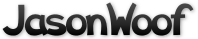Got questions, comments, patches, etc.? Contact Jason Woofenden
diff --git a/draw.h b/draw.h
index 43a57bf..17d2c89 100644 (file)
--- a/draw.h
+++ b/draw.h
@@ -1,35 +1,64 @@

-#define FG(dc, col)  ((col)[(dc)->invert ? ColBG : ColFG])
-#define BG(dc, col)  ((col)[(dc)->invert ? ColFG : ColBG])
+struct _XCol {
+       unsigned long rgb;
+};
+typedef struct _XCol Col;

-enum { ColBG, ColFG, ColBorder, ColLast };
+struct _XFont {
+       int ascent;
+       int descent;
+       unsigned int h;
+       XFontSet set;
+       XFontStruct *xfont;
+};
+typedef struct _XFont Fnt;

-typedef struct {
-       int x, y, w, h;
-       Bool invert;
+typedef struct _XDraw Draw;
+struct _XDraw {
+       unsigned int w, h;
Display *dpy;
+       int screen;
+       Window win;
+       Drawable drawable;
GC gc;
-       Pixmap canvas;
-       struct {
-               int ascent;
-               int descent;
-               int height;
-               int width;
-               XFontSet set;
-               XFontStruct *xfont;
-       } font;
-} DC;  /* draw context */
-
-void drawrect(DC *dc, int x, int y, unsigned int w, unsigned int h, Bool fill, unsigned long color);
-void drawtext(DC *dc, const char *text, unsigned long col[ColLast]);
-void drawtextn(DC *dc, const char *text, size_t n, unsigned long col[ColLast]);
-void eprintf(const char *fmt, ...);
-void freedc(DC *dc);
-unsigned long getcolor(DC *dc, const char *colstr);
-DC *initdc(void);
-void initfont(DC *dc, const char *fontstr);
-void mapdc(DC *dc, Window win, unsigned int w, unsigned int h);
-void resizedc(DC *dc, unsigned int w, unsigned int h);
-int textnw(DC *dc, const char *text, size_t len);
-int textw(DC *dc, const char *text);
+       Col *fg;
+       Col *bg;
+       Fnt *font;
+};
+
+typedef struct {
+       unsigned int w;
+       unsigned int h;
+       int xOff;
+       int yOff;
+} TextExtents;
+
+/* Drawable abstraction */
+Draw *draw_create(Display *dpy, int screen, Window win, unsigned int w, unsigned int h);
+void draw_resize(Draw *draw, unsigned int w, unsigned int h);
+void draw_free(Draw *draw);
+
+/* Fnt abstraction */
+Fnt *draw_font_create(Draw *draw, const char *fontname);
+void draw_font_free(Draw *draw, Fnt *font);
+
+/* Colour abstraction */
+Col *draw_col_create(Draw *draw, const char *colname);
+void draw_col_free(Draw *draw, Col *col);
+
+/* Drawing context manipulation */
+void draw_setfont(Draw *draw, Fnt *font);
+void draw_setfg(Draw *draw, Col *col);
+void draw_setbg(Draw *draw, Col *col);
+
+/* Drawing functions */
+void draw_rect(Draw *draw, int x, int y, unsigned int w, unsigned int h, Bool filled, Bool empty, Bool invert);
+void draw_text(Draw *draw, int x, int y, unsigned int w, unsigned int h, const char *text, Bool invert);
+
+/* Map functions */
+void draw_map(Draw *draw, int x, int y, unsigned int w, unsigned int h);
+
+/* Text functions */
+void draw_getextents(Draw *draw, const char *text, unsigned int len, TextExtents *extents);
+